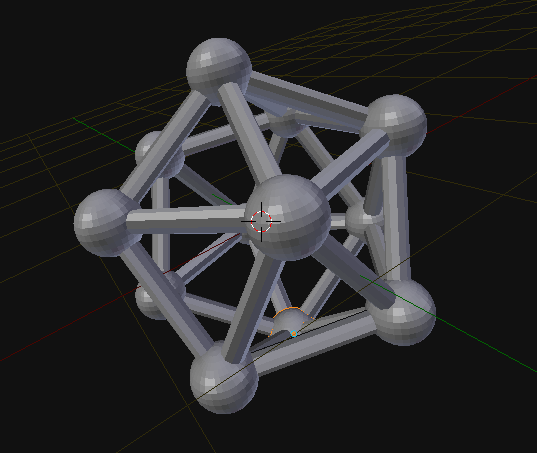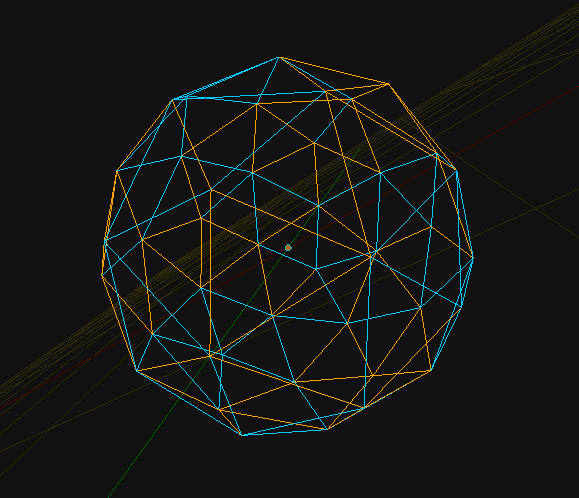# Script that does split to all edges of mesh into individual edge

Hi all,

I’ve been searching for a way to split mesh edges into each individual edge. I tried so many ways, but failed.

If I were to manually do it:

1. Select a mesh
2. Go to Edit mode.
3. Delete all faces, but Face Only.
4. I left with edges.
5. I manually select edge and press Y, this is exactly what I am trying to do. But I want it to repeat continuosly until all edges are separate individual edge.

The closest script to what I am trying to do is this:

########

import bpy

obj = bpy.context.active_object
bpy.ops.object.mode_set(mode=‘OBJECT’)

for i in obj.data.edges:
if i.crease > 0.0:
i.select = True

bpy.ops.object.mode_set(mode=‘EDIT’)

########

But in that case, it only select edges with Crease bigger than 0.

I tried to plug this somewhere, but does not seem to work:
bpy.ops.mesh.split()

I am not programmer per se, so really need help for this. Thank you.

this script will do something like that, not with operators but with mesh.from_pydata
this could be done the other way too, with operators…

``````
###############################
# create meshes from edges... #
###############################

import bpy
obj = bpy.context.object
edg = obj.data.edges
ver = obj.data.vertices

for e in edg:
v0 = ver[e.vertices].co
v1 = ver[e.vertices].co
mes = bpy.data.meshes.new('mesh.000')
mes.from_pydata([v0, v1], [(0, 1)], [])
mes.update()
new = bpy.data.objects.new('edge.000', mes)

``````

you can set origin to geometry in one go for all pieces if you need to Ctrl+Alt+Shift+C

i like liero’s approach there

``````
import bpy

obj = bpy.context.active_object

bpy.ops.object.mode_set(mode='EDIT')
bpy.ops.mesh.delete(type='ONLY_FACE')

bpy.ops.object.mode_set(mode='OBJECT')
for indx in range(len(obj.data.edges)):
print(indx)

obj.data.edges[indx].select = True

bpy.ops.object.mode_set(mode='EDIT')
bpy.ops.mesh.split()

bpy.ops.object.mode_set(mode='OBJECT')
obj.data.edges[indx].select = False

``````

would be the operator way

liero,
thank you so much! you solved it. I’ve been having headache for hours wondering why the script does not work. no matter how I modify it.

zeffii,
I was reading your script and trying to do this but failed. I don’t know why I can’t use bpy.ops.mesh.split()

Ok, I will post what I am trying to do later. cheers, you guys are fantastic, I love Blender.

Thank you again Liero and Zeffii. I am still wonder why I can’t use bpy.ops.mesh.split() like if I were to do it manually.

Anyhow, it does the job:

You guys could probably create script that does everything I wrote on that blogI am reading both scripts many times. Now, I got it. I will mark this one as solved. Thanks again.

not sure what you mean, because if you invoke bpy.ops.mesh.split() from the console while in edit mode with one edge selected, it does the same as when you press Y. However, if you want to select an edge using a script, you must switch to ‘OBJECT’ mode first. Check my code above closely. it switches in and out of object mode / edit mode

``````
import bpy

obj = bpy.context.active_object

bpy.ops.object.mode_set(mode='EDIT')
bpy.ops.mesh.delete(type='ONLY_FACE')

bpy.ops.object.mode_set(mode='OBJECT')
for indx in range(len(obj.data.edges)):
print(indx)
obj.data.edges[indx].select = True

bpy.ops.object.mode_set(mode='EDIT')
bpy.ops.mesh.split()

bpy.ops.object.mode_set(mode='OBJECT')
obj.data.edges[indx].select = False

bpy.ops.object.editmode_toggle()
bpy.ops.mesh.select_all(action='TOGGLE')
bpy.ops.object.editmode_toggle()
bpy.ops.object.convert(target='CURVE', keep_original=False)

bpy.context.active_object.data.use_fill_front = False
bpy.context.active_object.data.use_fill_back = False
bpy.context.active_object.data.extrude = 0.03
bpy.context.active_object.data.bevel_resolution = 5
bpy.context.active_object.data.bevel_depth = 0.04

``````

this will give you that bucky ball.

and this makes the atoms too

``````
import bpy

# while in object mode
obj = bpy.context.active_object

stored_verts = []
for vert in bpy.context.active_object.data.vertices:
stored_verts.append(vert.co)

for i in stored_verts:
print(i)

bpy.ops.object.mode_set(mode='EDIT')
bpy.ops.mesh.delete(type='ONLY_FACE')

bpy.ops.object.mode_set(mode='OBJECT')
for indx in range(len(obj.data.edges)):
print(indx)
obj.data.edges[indx].select = True

bpy.ops.object.mode_set(mode='EDIT')
bpy.ops.mesh.split()

bpy.ops.object.mode_set(mode='OBJECT')
obj.data.edges[indx].select = False

bpy.ops.object.editmode_toggle()
bpy.ops.mesh.select_all(action='TOGGLE')
bpy.ops.object.editmode_toggle()
bpy.ops.object.convert(target='CURVE', keep_original=False)

bpy.context.active_object.data.use_fill_front = False
bpy.context.active_object.data.use_fill_back = False
bpy.context.active_object.data.bevel_resolution = 3
bpy.context.active_object.data.bevel_depth = 0.068

bpy.ops.object.mode_set(mode='OBJECT')

for i in stored_verts:
print(i)

for coordinate in stored_verts:
ring_count=16,
size=0.19,
location=coordinate)

``````

both for scripts, consider that they assume the 3dcursor being at the origin of the scene (0,0,0)additional edge selection code examples can be found here :
selecting-edges-using-some-criteria.html (includes example of forcing edge select mode)